# DAV Class 7 Maths Chapter 12 Worksheet 4 Solutions

The DAV Maths Class 7 Solutions and DAV Class 7 Maths Chapter 12 Worksheet 4 Solutions of Data Handling offer comprehensive answers to textbook questions.

## DAV Class 7 Maths Ch 12 WS 4 Solutions

Question 1.
A bicycle shop owner sold the following number of bicycles each day in a particular week. Present this information in the form of a bar graph.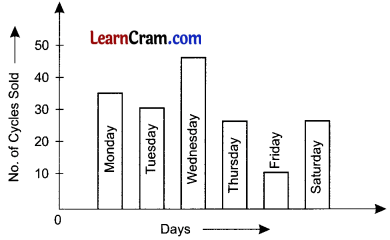Question 2.
The number of adults in a village according to their level of education are given in the graph.
(a) What does the bar graph represent?
(b) How many uneducated adults are there?
(c) How many adults are educated upto (i) Primary level (ii) High school level?(a) The above bar graph represent the status of education level of adults,
(c) (i) 900 (ii) 700.Question 3.
Draw bar graph for the following data.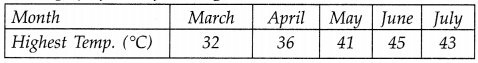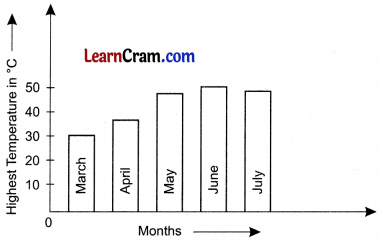Question 4.
Study the bar graph and answer the questions that follow: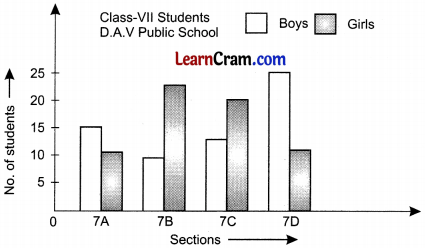(a) What does the bar graph represent?
(b) In which two sections are the number of girls equal ?
(c) Which section has the highest number of girls?
(d) How many sections have more number of girls than boys ?
(a) The bar graph represents the number of boys and girls in 4 sections of class-VII of D.A.V. Public School.
(b) 7 A and 7 D
(c) 7 B
(d) 2(7B and 7C).

Question 5.
The following data gives the number of Mathematics and Science books bought by a library during different years.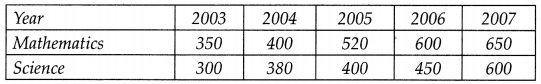Draw a double bar graph.Question 6.
The following data gives the maximum and minimum temperature (in °C) of the cities on a particular day. Plot a double bar graph from this data and answer the following questions: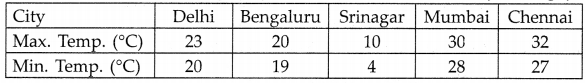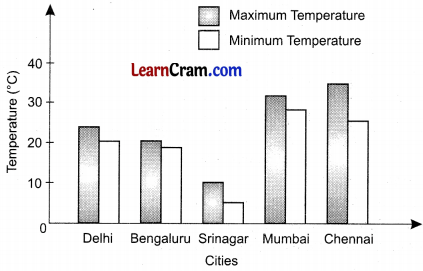(a) Srinagar shows the maximum difference in day’s temperature.
(b) Bengaluru shows the minimum difference in day’s temperature.
(c) Chennai is the hottest city and Srinagar is the coldest city.

### DAV Class 7 Maths Chapter 12 Value Based Questions

Question 1.
A group of ten students visited a blind school. They wanted to help the blinds by donating some money. They donated ₹ 120, ₹ 125, ₹ 100, ₹ 50, ₹ 75, ₹ 100, ₹ 150, ₹ 100, ₹ 75, ₹ 85 for this purpose
(i) Calculate the mean, median and mode of the above data of donation.
(ii) What values of the students are depicted here?
(i) Mean = $$\frac{120+125+100+50+75+100+150+100+75+85}{10}=\frac{980}{10}$$
= 98

Median: Arranging the data in ascending order, we have
₹ 50, ₹ 75, ₹ 75, ₹ 85, ₹ 100, ₹ 100, ₹ 100, ₹ 120, ₹ 125, ₹ 150
Two middle most observations are ₹ 100 and ₹ 100.
Median = $$\frac{\text { Sum of two middle most observations }}{2}$$
= $$\frac{100+100}{2}$$ [Here, median is the average (mean) of two middle most observation (5th and 6th observation)]
= ₹ 100
Mode: Arranging the data in ascending form, we have ₹ 50, ₹ 75, ₹ 75, ₹ 85, ₹ 100, ₹ 100, ₹ 100, ₹ 120, ₹ 125, ₹ 150 Here, ₹ 100 occurs most frequently (3 times).
So, the mode is ₹ 100.

(ii) Values: Students are caring and helpful. They helped the blind school by donating money.

Question 2.
A survey was conducted on the mode of transport used by 30 students of a Class-VI A for coming to school. It was found that 12 students came by school bus, 3 students by rickshaw, 2 by cycle, 8 by vans. Also 5 children did car pooling in Rahul’s car.
(i) Draw a bar graph for the above data.
(ii) Write one advantage of car pooling.
(iii) What value of the five children doing car pooling is depicted here?